Function Repository Resource:

# RollingCurve

Compute a rolling curve

Contributed by: Wolfram Staff (original content by Alfred Gray)
 ResourceFunction["RollingCurve"][c,r,h,t0,t] gives the parametrized curve traced out by a point P attached to a circle of radius r rolling along a plane curve c parametrized by variable t. The distance from P to the center of the rolling circle is h, and t0 is the point of the curve at which the circle starts rolling. ResourceFunction["RollingCurve"][c,r,t0,t] takes the distance from the tracing point to the center of the rolling circle to be Abs[r].

## Details

A rolling curve is also referred to as a roulette.
Rolling curves have been extensively studied for many applications in mechanical engineering.

## Examples

### Basic Examples (3)

Define the parametric equations for an ellipse:

 In:=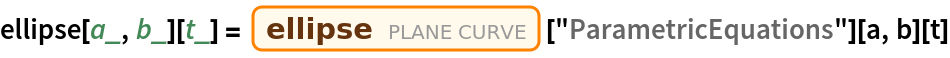Out=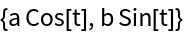Compute the rolling curve of the ellipse:

 In:=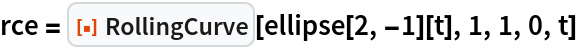Out=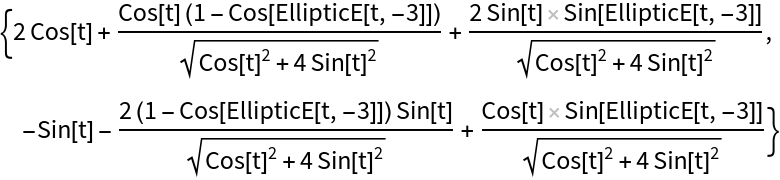Plot the rolling curve:

 In:=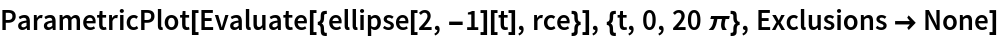Out=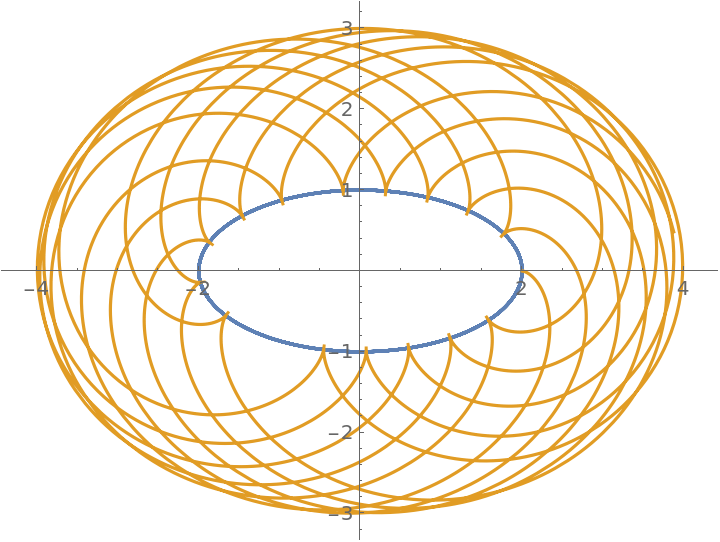### Scope (4)

Parametric equations for a circle:

 In:=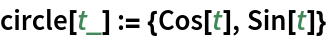The epitrochoid is the rolling curve of a circle if the rolling circle is outside the original circle:

 In:=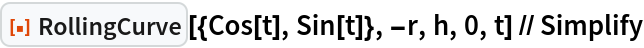Out=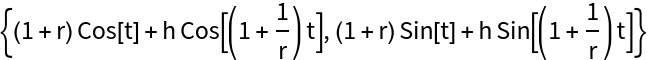The epicycloid is obtained if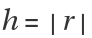:

 In:=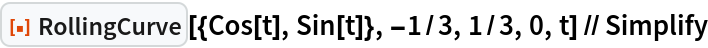Out=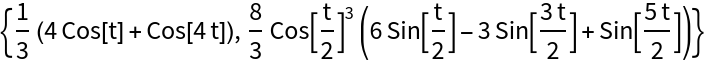An equivalent way to get the epicycloid:

 In:=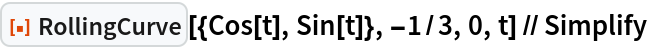Out=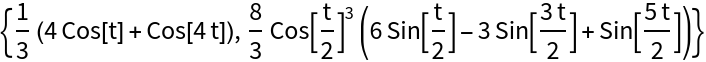### Properties and Relations (5)

Define the parametric equations of a parabola:

 In:=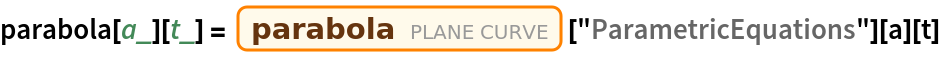Out=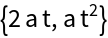Define the rolling curve of the parabola:

 In:=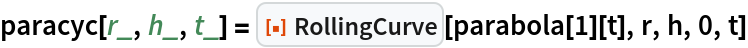Out=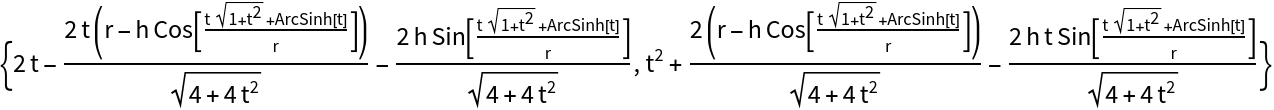When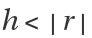(the curtate case), the rolling curve is smooth:

 In:=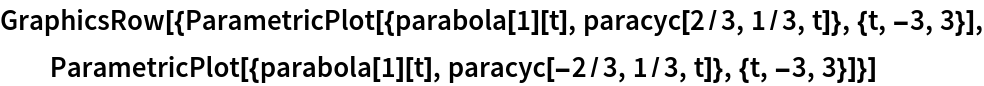Out=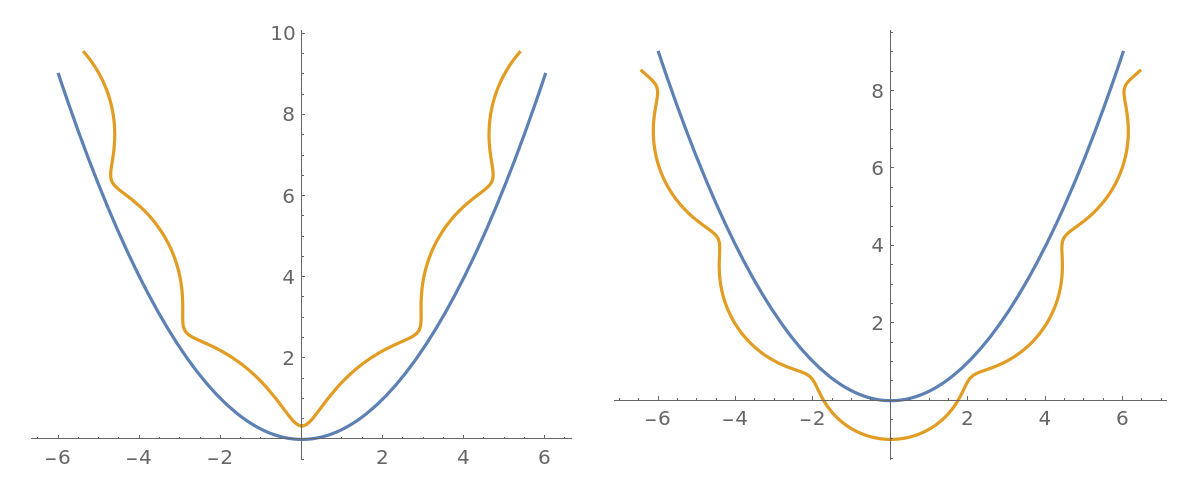When(the cycloidal case), the rolling curve has cusps:

 In:=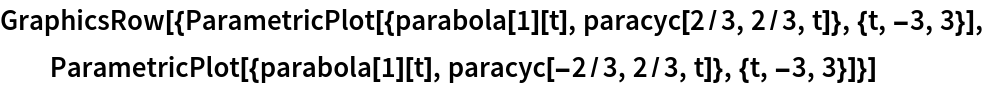Out=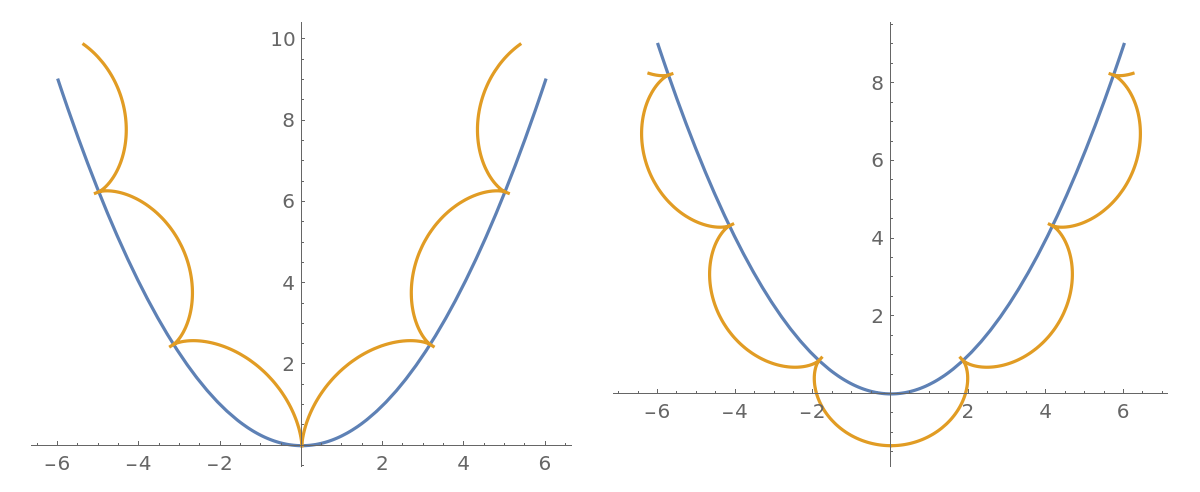When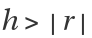(the prolate case), the rolling curve has self-intersection points:

 In:=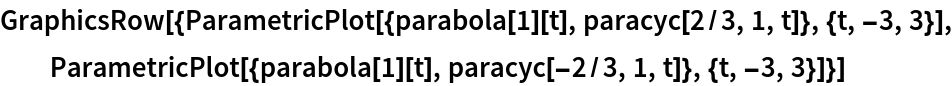Out=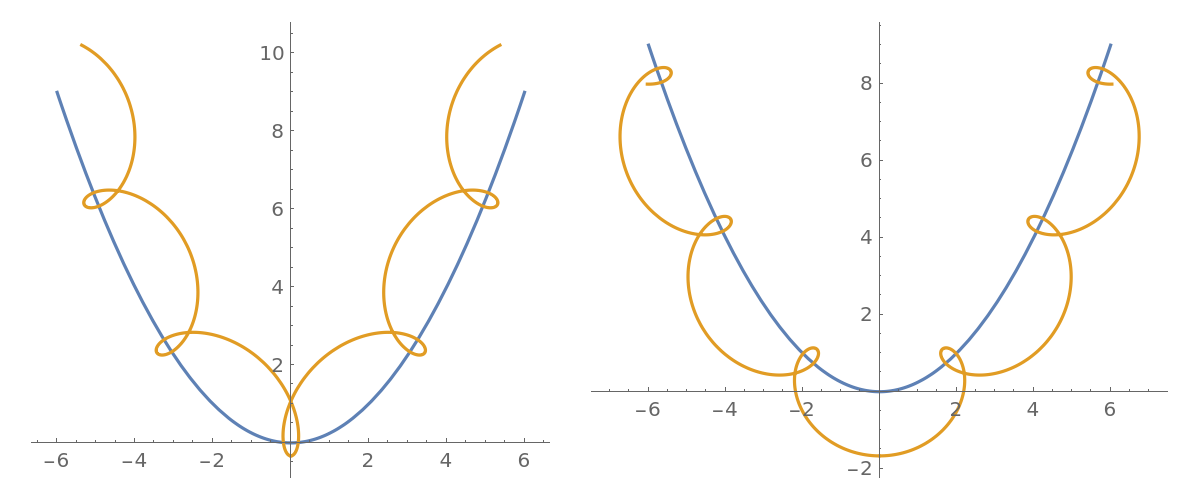### Neat Examples (4)

Define the parametric equations of a trochoid:

 In:=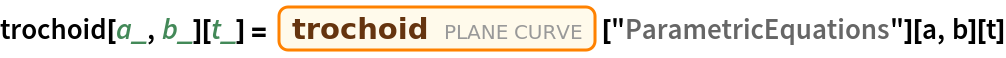Out=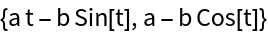Compute the parallel curve of the trochoid:

 In:=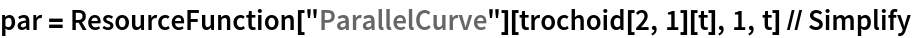Out=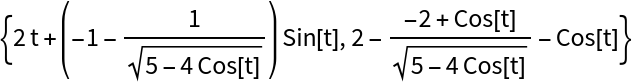Compute the rolling curve of the trochoid:

 In:=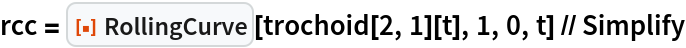Out=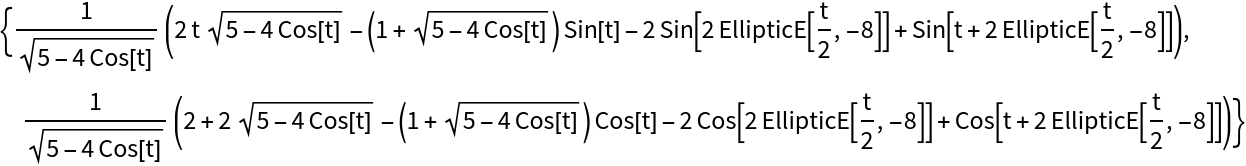Create a Manipulate that rolls a circle along the curve:

 In:=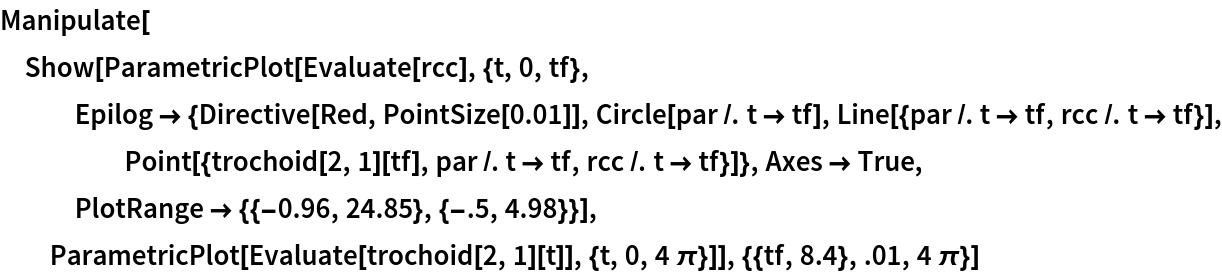Out=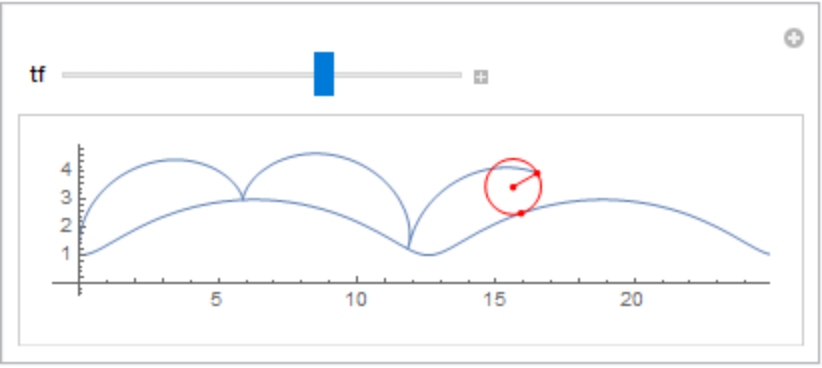Enrique Zeleny

## Version History

• 1.0.1 – 29 March 2021
• 1.0.0 – 28 February 2020